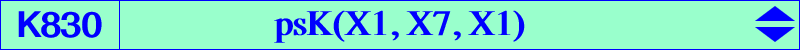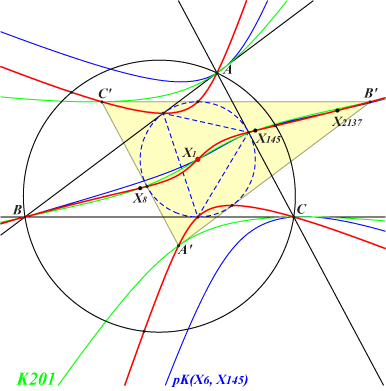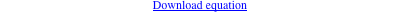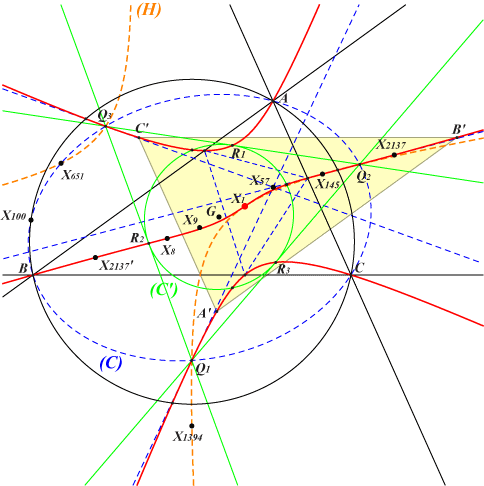too complicated to be written here. Click on the link to download a text file.X(1), X(8), X(145), X(2137) reflections of A, B, C, X(2137) about X(1) vertices of the intouch triangle their reflections about X(1) other points belowK830 is a central psK with center X(1). See the related K201, a central pK with center X(1) and also K831, a central focal cubic with center X(1). K830 meets the line at infinity at the same points as K1086 = pK(X6, X145) and the circumcircle at the same points as pK(X6, P) where P = X(14923) is the common point of the lines 1,88 - 2,3057 - 4,8 - 5,1145 - etc. K1314 is the image of K830 under SS{a → a^2}.The tangents at A, B, C meet at X(9), the pseudo-isopivot of the psK and the center of the circum-conic (C) with perspector X(1). K830 meets (C) again at three points Q1, Q2, Q3 with tangents concurrent at X(57). It follows that the polar conic (H) of X(57) passes through these three points and also X(1), X(145), X(651), X(1394). K830 meets the sides of triangle Q1Q2Q3 again at their midpoints R1, R2, R3 and the Steiner inellipse (C') of triangle Q1Q2Q3 has center G. Obviously, K830 passes through the reflections of all these points about X(1). K830 is transformed into the same cubic, namely psK(X1, X7, X2), under either X(1)-isoconjugation or X(7)-Ceva conjugation.